# Algebra - Basics and Visual Introduction

Go back to  'Algebra Basics'

## A simple way to understand the basics of Algebra

You’ll hear the works variable, constant, expression, monomial, binomial and polynomial frequently when dealing with algebra.

Here’s a quick glossary and definitions of Algebraic terms: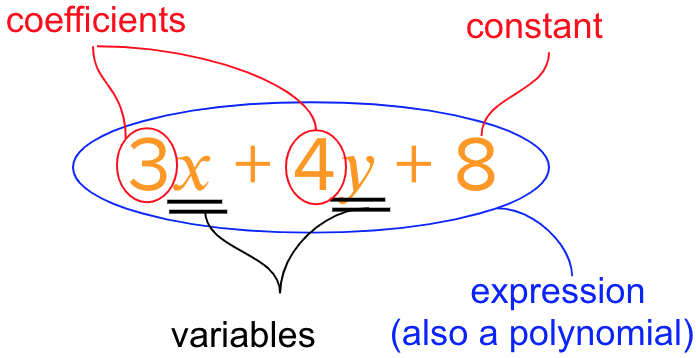### Variable - definition and example

Variables are symbols that stand for unknown numbers or a specific range of numbers.

These symbols will generally be lower case letters like $$x,\,y,\,z,\,m,\,a,$$ etc.
E.g. A square with unknown side length can be said to have sides of length $$x$$.
E.g. Even numbers can be represented as $$2x$$ where $$x$$ can take values like 1, 2, 3, 4…

### Coefficients - definition and example

Coefficients are numbers that are multiplied with a variable.

E.g. $$2x$$ - here the number 2 is the coefficient of the variable $$x.$$
$$2x$$ means the variable $$x$$ is multiplied by 2.
Coefficients can be positive or negative. They can be whole numbers or decimals or fractions.

### Constants - definition and example

Numbers that appear on their own, without a variable multiplied to them, are called constants.

E.g. In $$3x - 5$$ the variable is $$x,\,3$$ is the coefficient of $$x$$ and $$- 5$$ is the constant.

### Algebraic Expressions - definition and example

When we combine variables (with or without coefficients written) and constants into a mathematical statement, this statement is called an algebraic expression.

E.g. If a variable $$x$$ is multiplied by 6 and then 9 is added to it, we get $$6x + 9.$$
$$6x + 9$$ is an algebraic expression.

### Term - definition and example

A term is a variable and it’s coefficient on its own or a constant on its own. Terms are separated in an algebraic expression by the addition or subtraction operators.

E.g. The algebraic expression $$6x + 9$$ has two terms: 6𝑥 and 9.
E.g. The algebraic expression $$-3x$$ is just a single term in itself.

### Polynomial - definition and example

All algebraic expressions which are a combination of variables and constants are called polynomials. However, to be a polynomial an algebraic expression cannot have a variable in the denominator nor can the exponent of the variable be negative or a fractional exponent.

E.g. $$5x^2 - 3$$ is a polynomial.
E.g. \begin{align}\left( {\frac{5}{{x^2 }}} \right) - 3 \end{align} is not a polynomial because the variable $$x^2$$ is in the denominator
E.g. $$5x^{-2} - 3$$ is not a polynomial because the exponent of the variable is $$-2.$$

### Monomial - definition and example

Algebraic expressions with exactly one term are called monomials. (mono = 1)

E.g. $$5x$$ is a monomial because it has exactly one term. But $$5x - 3$$ is not a monomial because it has two terms $$(5x \text{ and } -3).$$

### Binomial - definition and example

Algebraic expressions with exactly two terms are called binomials. (bi = two)

E.g. $$5x -3$$ is a binomial because it has two terms $$(5x \text{ and } -3).$$
E.g. $$9y - 4x$$ is also a binomial because it has two terms $$(9y \text{ and } -4x).$$

The word Algebra has Arabic roots and comes from the word “al-jabr” which means “the reunion of broken parts”.

## Rules of working with variables

\begin{align} 3y &= y + y + y \\\\ y^3 &= y \times y \times y \\\\ 2y^3 &= y^3 + y^3 \\ &= (y \times y \times y) + (y \times y \times y) \end{align}

## Thinking of algebra in terms of visual patterns

We come across many patterns in our everyday life. Algebra is a tool to mathematically represent those patterns. So, it is best to start algebra by deciphering simple patterns. Here is a good example of how patterns can be the first step to learning algebra

Example 1

Problems like the one given below, help convert simple patterns into mathematical representations.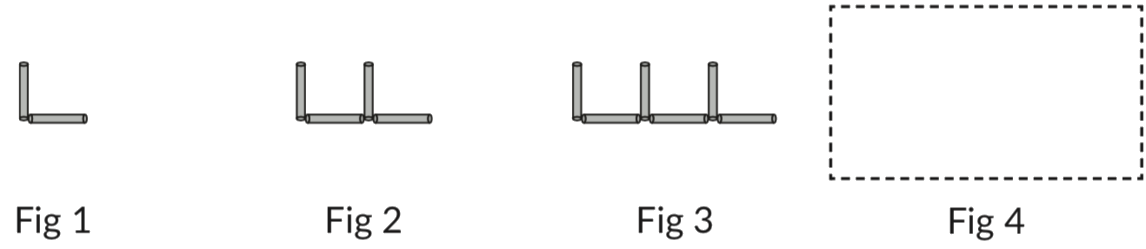What’s the pattern here? How many sticks will be there in figure 4?

• We see that the number of “L”s in the figure increases by 1 in each figure.
• So, Fig 1 has 1 L, Fig 2 has 2 Ls and so on…
• So, Fig 4 will have 4 L
• Since each L has two sticks, figure 4 will have $$2 \times 4 = 8$$ sticks.
• What about the 100th figure? It will have $$2 \times 100 = 200$$ sticks.

Thinking about algebra as patterns allow us to answer such questions without actually creating the figure.

Example 2

Look at the figures given in the figures below.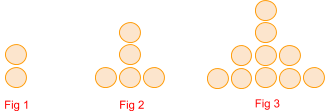What’s the pattern here? How many circles will be there in figure 4?

• Rearranging the dots helps us see a pattern clearly. We can see that the circles form a square pattern with one additional dot.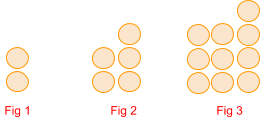• Fig 1 has $$1 + 1 = 2$$ dots. Can be written as $$12 + 1 = 2.$$
• Fig 2 has $$4 + 1 = 5$$ dots. Can be written as $$22 + 1 = 5.$$
• Fig 3 has $$9 + 1 = 10$$ dots. Cna be written as $$32 + 1 = 10.$$

So, the pattern in fig 4 will be $$4^2 + 1 = 17.$$

The pattern in any subsequent figure number, let’s say $$n,$$ will be $$n^2 + 1.$$

## Thinking of algebra using everyday language

Through examples like the ones listed below, we see that the letters can be used to stand-in for unknown numbers or sets of numbers. This is how students should see the variables.

1. Raman has 8 books more than Rani. If Rani has x books, then Raman has $$\underline {\;\;\;\;\;x + 8\;\;\;\;\;}$$ books.

2. Mallika is 5 years older than Suman. If Suman is y years old, then Mallika is ________ years old

3. India scored 3 goals more than Pakistan. If Pakistan scored y goals, then India scored________ goals.

4. The current price of petrol is Rs p. It will increase by Rs 3 next month. What would  be its new price next month?________

## Building Algebraic Statements

Converting a verbal statement into mathematical form by using a variable is an important skill. Problems like the ones shown here can be employed to build that skill.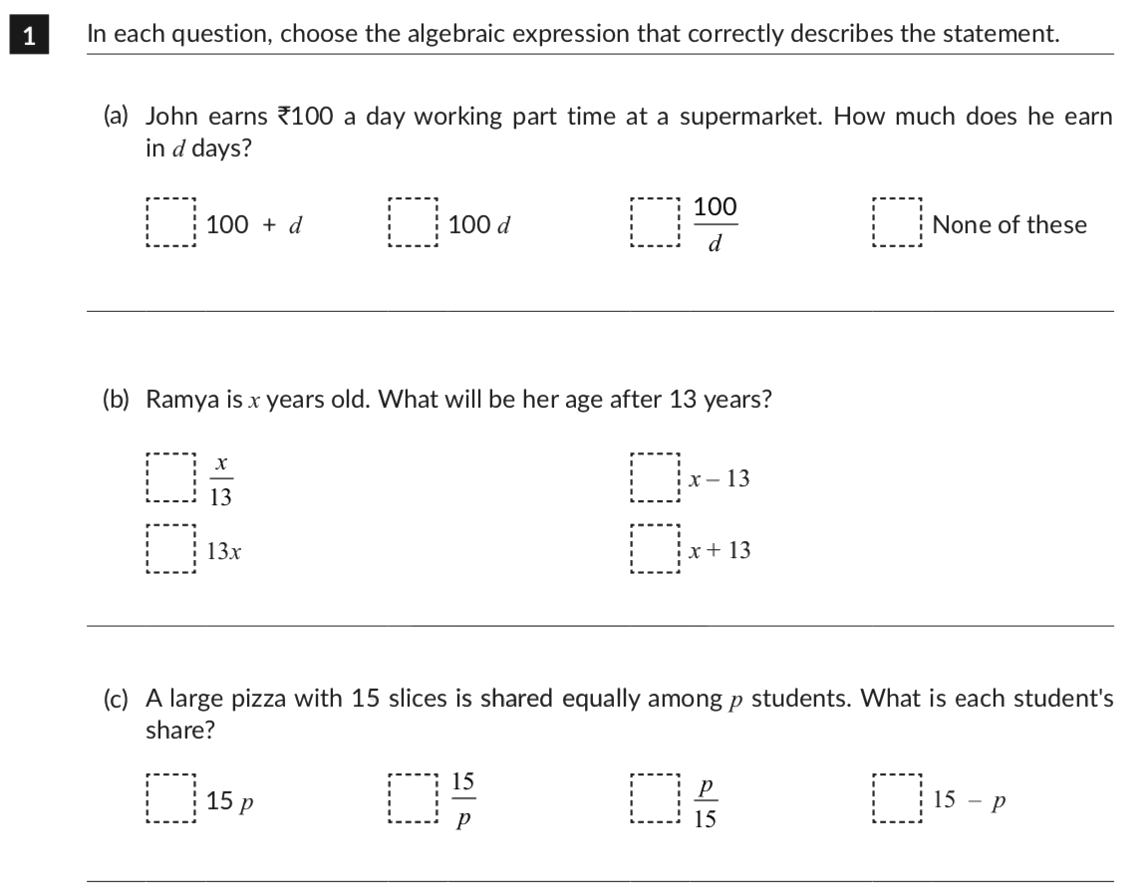Next skill is to interpret and rewrite abstract statements algebraically.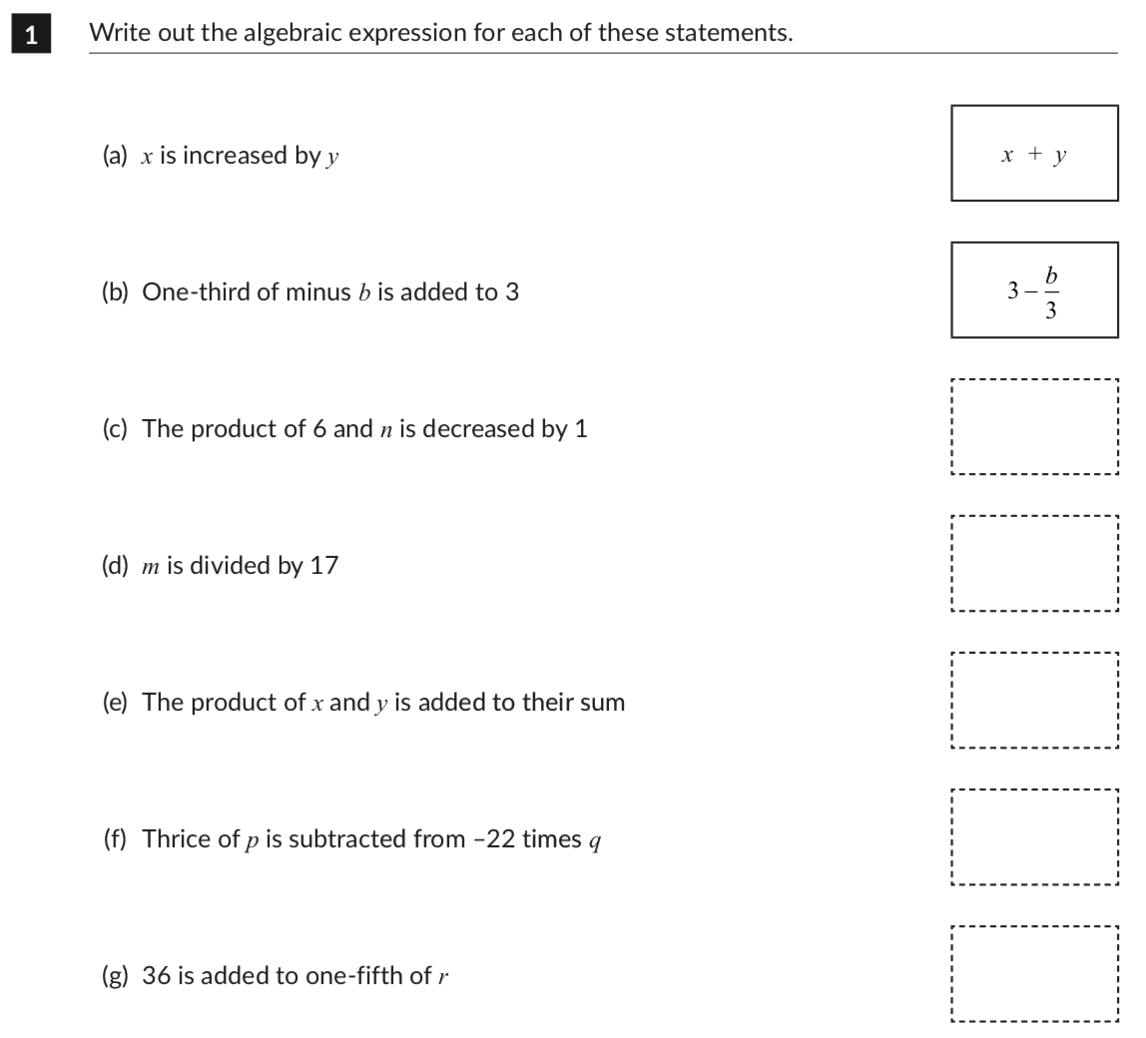## Introducing the concept of substitution

Algebraic Expressions can be evaluated by assigning certain specific values to the variables. Depending on what value we assign to a variable the final value of the expression will change.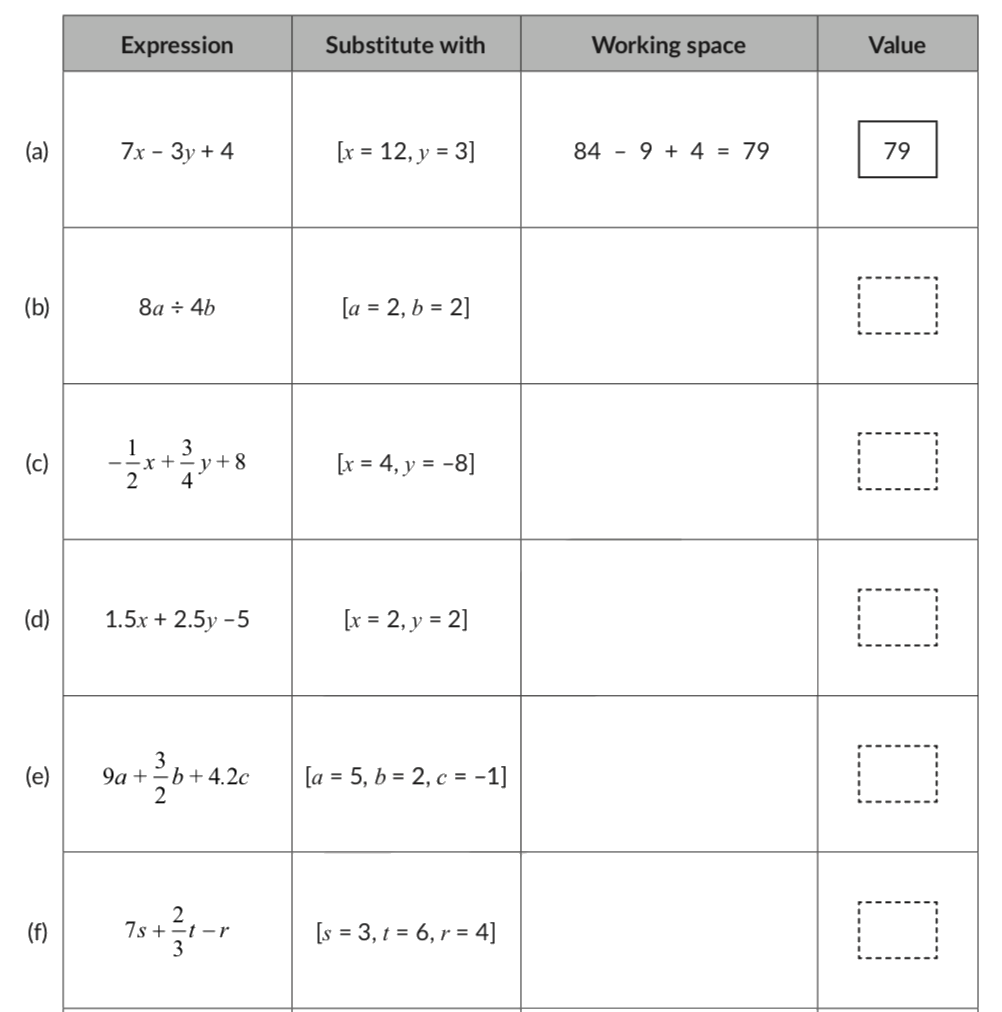## Tips and Tricks to understand the Basics of Algebra

• A helpful tip to get children comfortable with algebra is presenting many everyday examples and asking children to describe that as an algebraic expression:
E.g. I bought 3 packs of biscuits. How much did I spend?
This statement seems incomplete as the price of biscuits is unknown. This is algebra. Children should be able to state this as “The amount spent is $$3x$$” where $$x$$ is the price of the biscuits.
E.g. Let’s buy two more presents that the number of people invited to the party.
The number of gifts to be bought will be $$x + 2$$ where $$x$$ is the number of people invited.
• Tip: Students may incorrectly believe that there is a fixed convention to using variables. E.g. if an expression has a variable, then that variable must be $$“x”.$$ This isn’t true. Ask children to build an expression with many different variables. E.g. $$“a + 3” \text{ or } “5m”,$$ etc.
• Help children notice that there can be certain letters that look like variables but may not be so. In higher grade math they will encounter numerical constants like the speed of light (denoted by $$c$$), pi ($$\pi$$), the base of the natural logarithm ($$e$$). These are constant and not variables even though are denoted by letters. So it is important to define if a letter denotes a variable or a constant.
• It’s best to visualise algebraic terms geometrically. E.g. $$x$$ can be visualised as a length, $$x^2$$ or $$xy$$ as an area and $$x^3$$ or $$xyz$$ as volume.